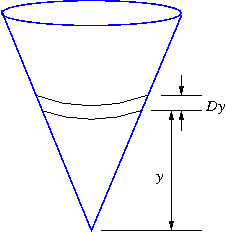Consider the volume of the region shown below, which shows a right circular cone with top radius 1 cm and height 5 cm. We have used the notation $Dy$ for $\Delta y$.Write a Riemann sum for the volume, using the strip shown and the variable $y$:
Riemann sum $= \Sigma$

Now write the integral that gives this volume:
volume $= \int_a^b\,$
where $a =$
and $b =$.

Finally, calculate the exact volume of the region, using your integral.
volume =
(include units)

You can earn partial credit on this problem.